# Implication

Implication or entailment is used in propositional logic and predicate logic to describe a relationship between two sentences or sets of sentences, in which one sentence or set of sentences is said to "lead to" or "imply" or "entail" the other sentence or set of sentences, and the other is said to "follow from" or be "derived from" or be "entailed by" or be "implied by" the former.

## Logical Implication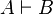$A \vdash B$

states that the set A of sentences logically entails the set B of sentences. It can be read as "B can be proven from A."

Definition: A logically entails B if, by assuming all sentences in A are true, and applying a finite sequence of inference rules to them (for example, those from propositional calculus), one can derive all sentences in B.

## Semantic Implication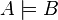$A \models B$

states that the set A of sentences semantically entails the set B of sentences.

Formal definition: the set A entails the set B if and only if, in every model in which all sentences in A are true, all sentences in B are also true. In diagram form, it looks like this:

We need the definition of entailment to demand that every model of A must also be a model of B because a formal system like a knowledge base can't possibly know the interpretations which a user might have in mind when they ask whether a set of facts (A) entails a proposition (B).

In pragmatics (linguistics), entailment has a different, but closely related, meaning.

If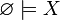$\varnothing \models X$ for a formula X then X is said to be "valid" or "tautological."

## Relationship between Semantic and Logical Implication

Ideally, semantic implication and logical implication would be equivalent. However, this may not always be feasible. (See Gödel's incompleteness theorem, which states that some languages (such as arithmetic) contain true but unprovable sentences.) In such a case, it is useful to break the equivalence down into its two parts:

A deductive system S is complete for a language L if and only if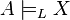$A \models_L X$ implies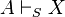$A \vdash_S X$: that is, if all valid arguments are provable.

A deductive system S is sound for a language L if and only if$A \vdash_S X$ implies$A \models_L X$: that is, if no invalid arguments are provable.

## Material Conditional

In propositional calculus, or logical calculus in mathematics, the material conditional or the implies operator is a binary truth-functional logical operator yielding the form

If a then c,

where a and c are statement variables (to be replaced by any meaningful indicative sentence of the language). In a statement of this form, the first term, in this case a, is called the antecedent and the second term, in this case c, is called the consequent. The truth of the antecedent is a sufficient condition for the truth of the consequent, while the truth of the consequent is a necessary condition for the truth of the antecedent.

The operator is symbolized using a right-arrow "→" (or sometimes a horseshoe "⊃"). "If A then B" is written like this: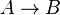$A \to B$

## Relationship with Material Implication

In many cases, entailment corresponds to material implication: that is,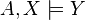$A, X \models Y$ if and only if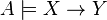$A \models X \to Y$ . However, this is not true in some many-valued logics.

Standard logic is two-valued, meaning that statements can be only true or false, and every statement is either true or false. So if a statement is not false it is true, and if it is not true it is false. In many-valued logics those conditions do not necessarily hold.

## Symbolization

A common exercise for an introductory logic text to include is symbolizations. These exercises give a student a sentence or paragraph of text in ordinary language which the student has to translate into the symbolic language. This is done by recognizing the ordinary language equivalents of the logical terms, which usually include the material conditional, disjunction, conjunction, negation, and (frequently) biconditional. More advanced logic books and later chapters of introductory volumes often add identity, Existential quantification, and Universal quantification.

Different phrases used to identify the material conditional in ordinary language include if, only if, given that, provided that, supposing that, implies, even if, and in case. Many of these phrases are indicators of the antecedent, but others indicate the consequent. It is important to identify the "direction of implication" correctly. For example, "A only if B" is captured by the statement

A → B,

but "A, if B" is correctly captured by the statement

B → A

When doing symbolization exercises, it is often required that the student give a scheme of abbreviation that shows which sentences are replaced by which statement letters. For example, an exercise reading "Kermit is a frog only if muppets are animals" yields the solution:

A → B, A - Kermit is a frog. B - Muppets are animals.

## Truth table

The truth value of expressions involving the material conditional is defined by the following truth table:

p q pq
F F T
F T T
T F F
T T T

## Comparison with other conditional statements

The use of the operator is stipulated by logicians, and, as a result, can yield some unexpected truths. For example, any material conditional statement with a false antecedent is true. So the statement "2 is odd implies 2 is even" is true. Similarly, any material conditional with a true consequent is true. So the statement, "If pigs fly, then Paris is in France" is true.

These unexpected truths arise because speakers of English (and other natural languages) are tempted to equivocate between the material conditional and the indicative conditional, or other conditional statements, like the counterfactual conditional and the material biconditional. This temptation can be lessened by reading conditional statements without using the words "if" and "then." The most common way to do this is to read A → B as "it is not the case that A and/or it is the case that B" or, more simply, "A is false and/or B is true." (This equivalent statement is captured in logical notation by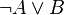$\neg A \vee B$, using negation and disjunction.)